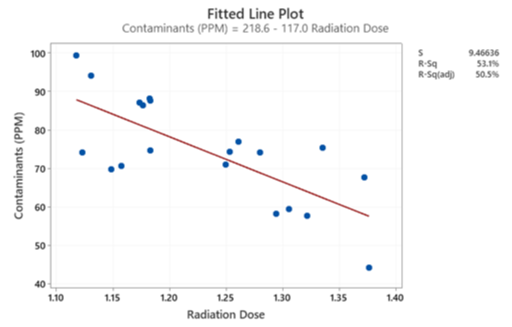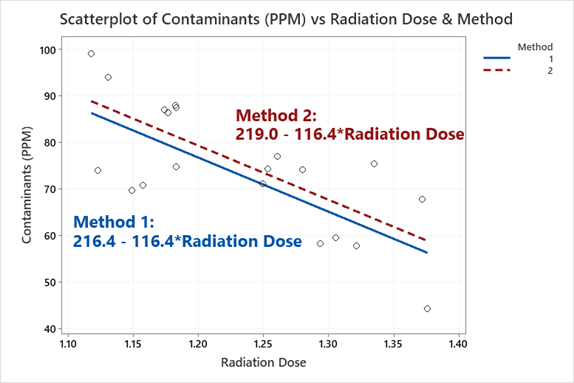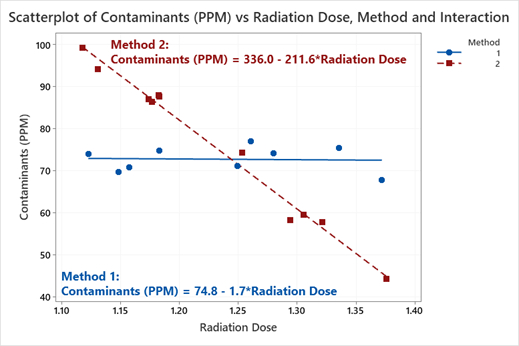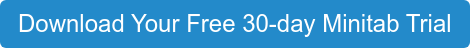Regression can pretty much do it all! But, most of the time, we like to think of a Regression problem as a best fitted line:

Predicted y = mx + b

The slope is denoted by m, which denotes the average change in y for every 1-unit increase in x. The y-intercept is denoted by b, the average outcome of y when x equals zero. In practical uses, we care more about the impact of x and focus on the slope.

In regression, the null hypothesis assumes the slope of the line equals zero. If the p-value is less than 0.05 then there is a significant main effect present and a relationship can be established. In this post, we will explore how the slope and intercept are impacted when adding categorical variables and interaction terms to a regression model.

## Regression: Best Fitted Line

Here is an example where radiation is used to sterilize products for medical device companies. The company is testing two methods of dosing with radiation, method 1 versus method 2. The dosage amount was also recorded. The goal is to find which method will keep the contaminants below 70 PPM without having to maximize dosage.With the model only including the continuous input of radiation dosage, there is an evident negative relationship and the maximum dosage is going to do the best job overall. The model is

Average PPM = 218.6 – 117.0*Dose

For every 1 full unit in radiation dosage the average number of contaminates decreases by 117 units. Since, our inferential space is between 1.1 and 1.4, it makes more sense to talk about 0.1-unit increase, which decreases contaminants by 11.7 PPM.

But the company wants to see if one of the methods works better to minimize the dosage amount and keep contaminants less than 70 PPM. First, let us explore the additive model, where we only account for the two main effects and no interaction.

## Regression Modeling with a Categorical Variable

A main effect is the pure impact the input has on the output, while keeping all other variables held constant. For example, in the section above, the main effect of radiation dose was a decrease of 117 PPM for every one unit increase in dose.

The null hypothesis for a categorical variable in Regression is treated as an ANOVA test of averages:

H0: μMethod1 = μMethod2

If the averages between the methods are different, then separate regression equations are created. Here the main effect of the categorical variable is comparable to the difference in the y-intercepts.There is a slight, if negligible, difference between the two methods.

Method 1 (PPM) = 216.4 – 116.4*Dose

Method 2 (PPM) = 219.0 – 116.5*Dose

Method 1 does slightly better than Method 2 because the y-intercept is less.

Because the interaction term was left out, Minitab assumes that the impact of dose is constant for both methods; therefore, the slopes of the lines are equal. There was a slight change in the slope from the Radiation Dose only model this difference is due to including the different methods on the impact of contaminants.

Main effects alone can be misleading, and it is always best practice to look at the interaction between the variables to test for differences in the slopes.

## Interaction Regression Model

With interactions we give the analysis more flexibility to identify more nuanced patterns, especially with different levels of a categorical variable. Instead of holding the slope of the radiation dose amount constant, the impact of radiation dose will be calculated for each method. The null assumes the slopes will be the same (β is often used to reference coefficients in the population):

H0: βMethod1 = βMethod2If Method 1 is used, there is no impact on contaminants by dosage amount and contaminants stay around 74 PPM on average, the slope is close to zero. But, if Method 2 is used we can increase dosage to 1.3 and get about 60 PPM on average, which is below the limit of 70 PPM.

If the company wants consistency, Method 1 is best and keep the dosage low. If the company wants to reduce contaminants below 70 PPM, then Method 2 at about 1.3 Radiation Dose is best. With the interaction, there are multiple optimal solutions that analysts should explore. Interactions give analysts the ability to make informed dynamic decisions.

## How Categorical Variables and Interaction Terms Impact a Regression Model

Minitab’s Regression menu allows for easy to interpret regression output and features but understanding the core concepts behind regression analysis can empower analysts to make correct decisions. Categorical terms and interaction terms have many implications in our analyses and they should always be fully vetted and understood.

### Want to Try Analysis Like This Yourself?